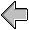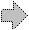# Complete Program from Figure 14

```(define Basic-Shapes
(unit (import)
(export Shape Rectangle Circle Translated)

(define Shape (interface () draw))

(define Rectangle
(class* object% (Shape) (width height)
(public
[draw (lambda (dc x y)
(send dc draw-rectangle x y width height))])
(sequence (super-init))))

(define Circle
(public
[draw (lambda (dc x y)
(send dc draw-ellipse
(sequence (super-init))))

(define Translated
(class* object% (Shape) (orig-shape dx dy)
(public
[draw (lambda (dc x y)
(send orig-shape draw
dc (+ x dx) (+ y dy)))])
(sequence (super-init))))))

(define Union-Shape
(unit (import Shape)
(export Union)

(define Union
(class* object% (Shape) (left right)
(public
[draw (lambda (dc x y)
(send left draw dc x y)
(send right draw dc x y))])
(sequence (super-init))))))

(define Basic+Union-Shapes
(compound-unit
(import)
[US (Union-Shape (S Shape))])
(export(S Shape)
(S Rectangle)
(S Circle)
(S Translated)
(US Union))))

(define BB-Shapes
(unit (import Shape Rectangle Circle Translated Union)
(export BB-Shape BB-Rectangle BB-Circle
BB-Translated BB-Union
make-BB BB-left BB-top BB-right BB-bottom)

(define BB-Shape (interface (Shape) bounding-box))

(define-struct BB (left top right bottom))

(define BB-Rectangle
(class* Rectangle (BB-Shape) (width height)
(public
[bounding-box
(lambda () (make-BB 0 0 width height))])
(sequence (super-init width height))))

(define BB-Circle
(class* Circle (BB-Shape) (r)
(public
[bounding-box
(lambda () (make-BB (- r) (- r) r r))])
(sequence (super-init r))))

(define BB-Translated
(class* Translated (BB-Shape) (shape dx dy)
(public
[bounding-box
(lambda ()
(let ([pre-bb (send shape bounding-box)])
(make-BB (+ (BB-left pre-bb) dx)
(+ (BB-top pre-bb) dy)
(+ (BB-right pre-bb) dx)
(+ (BB-bottom pre-bb) dy))))])
(sequence (super-init shape dx dy))))

(define BB-Union
(class* Union (BB-Shape) (left right)
(public
[bounding-box
(lambda ()
(let ([left-bb (send left bounding-box)]
[right-bb (send right bounding-box)])
(make-BB (min (BB-left left-bb) (BB-left right-bb))
(min (BB-top left-bb) (BB-top right-bb))
(max (BB-right left-bb) (BB-right right-bb))
(max (BB-bottom left-bb) (BB-bottom right-bb)))))])
(sequence (super-init left right))))))

(define Basic+Union+BB-Shapes
(compound-unit
(import)
[BS (BB-Shapes (S Shape)
(S Rectangle)
(S Circle)
(S Translated)
(S Union))])
(export (S Shape)
(BS BB-Shape)

;rename BS's BB-Rectangle to Rectangle, etc.:
(BS (BB-Rectangle Rectangle))
(BS (BB-Circle Circle))
(BS (BB-Translated Translated))
(BS (BB-Union Union))

(BS make-BB)
(BS BB-left)
(BS BB-top)
(BS BB-right)
(BS BB-bottom))))

(define Color-Shape
(unit (import Shape)
(export C-Shape)

(define C-Shape
(class* Shape () args
(rename
[super-draw draw])

(public
[color "BLACK"]
[change-color
(lambda (c)
(set! color c))]
[draw
(lambda (dc x y)
(let ([old-color (send dc get-color)])
(send dc set-color color)
(super-draw dc x y)
(send dc set-color old-color)))])
(sequence
(apply super-init args))))))

(define Basic+Union+BB+Color-Shapes
(compound-unit
(import)
[CR (Color-Shape (S Rectangle))]
[CC (Color-Shape (S Circle))]
[CT (Color-Shape (S Translated))]
[CU (Color-Shape (S Union))])
(export (S Shape)
(S BB-Shape)
(S make-BB)
(S BB-left)
(S BB-top)
(S BB-right)
(S BB-bottom)
(CR (C-Shape Rectangle))
(CC (C-Shape Circle))
(CT (C-Shape Translated))
(CU (C-Shape Union)))))

```figurein context contents